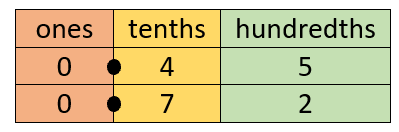# Math Worksheets Land

Math Worksheets For All Ages

# Math Worksheets Land

Math Worksheets For All Ages

# Comparing Decimals Worksheets

Being able to compare two decimal values is much different than standard whole number values. That is because the presence of a decimal point changes everything. It creates a gap between the integers that often is not fully understood by many students. This exists because not many students take the time to understand the concept of a whole unit value. The closer a decimal value is to a whole unit (1) the greater it is. These problems will work you through mastering this comparison and get you headed in right direction with this skill. A collection of lessons and worksheets that have students compare decimal values to the hundredths place.

### Aligned Standard: Grade 4 Fractions - 4.NF.7

• Answer Keys - These are for all the unlocked materials above.

### Homework Sheets

This section has you compare single decimals and order fractions from least to greatest. We also have you order the fractions in decreasing order.

• Compare Two HW 1 - Compare the digits, starting with the highest place value. The ones digits are the same. The tenths digits are different. The tenths digit in 0.567 is greater than the tens digit in 0.479.
• Least to Greatest HW 2 - Compare the digits, starting with the highest place value. The ones digits are the same. The tenths digits are different. Now compare from tenths digit. The bigger number is 5 on tenths place then 4 then 3 then 2.
• Decreasing Order HW 3 - Write the following decimals in decreasing order.

### Practice Worksheets

A mix of all the skills we touched on with the homework sheets.

• Practice 1 - Write the following decimal in order from least to the greatest.
• Practice 2 - Compare the decimals below by using >, <, or = symbols.
• Practice 3 - Write the following decimals in decreasing order.

### Math Skill Quizzes

I find these sheets to be a bit cramped as far as work area. Teachers asked me to keep it like this, I changed it once and got 5 emails that day on it.

• Quiz 1 - A mix of all the skills we have learned.
• Quiz 2 - Write the following decimals in decreasing order.
• Quiz 3 - Write the following decimal in order from least to the greatest.

### How to Compare Two Decimal Values

Learning decimals can be very tricky; especially when you must compare the value of decimal numbers with each other. In order to understand this, you need to understand the significance of the number 1; which, in this case, is a whole unit. All decimal numbers are part of a whole and 1 is the representation of that whole value.

Let's further understand this with an example of two decimal numbers 0.54 and 0.85. Here, you are provided with two decimal numbers with an equal number of digits. With the following steps below, you can easily compare two decimal numbers.

First, you will need to identify how closer each number is nearest to 1. Then the closest number to 1 will be the largest decimal number among the two. In this case, 0.85 is greater than 0.54 (0.85 > 0.54).

It is simpler to compare decimal numbers when the number of digits is the same. However, for decimal numbers having unequal number of digits, we need to look at the decimal places of the number. If the tenth place of both decimals is different. Then simply, the number with the largest tenths value will be greater. For example: 0.45 < 0.72. In this case, 7 is greater than 4, so the greater decimal number is 0.72.

Now that you have the basics, lets hammer home a true strategy that you can use each and every time you need to make this comparison.

Step 1 – Create Place Value Chart

Start by creating a simple place value chart that displays exactly the significance of each digit in a decimal value. It should look something like this for the values we just compared:Step 2 – Compare Digits from Greatest to Least

The greatest value is found furthest to the left. If the values are same, move to the next place to the right and compare those digits. In this case, we compare the ones column which are both 0. This means that we move one place to right (tenths) and compare those digits. At the tenths place 7 is greater that 4, this means that this decimal value (0.72) is the greater overall value.

Unlock all the answers, worksheets, homework, tests and more!
Save Tons of Time! Make My Life Easier Now

## Thanks and Don't Forget To Tell Your Friends!

I would appreciate everyone letting me know if you find any errors. I'm getting a little older these days and my eyes are going. Please contact me, to let me know. I'll fix it ASAP.